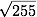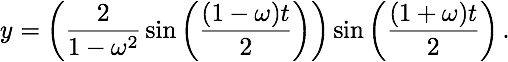# Forced vibration

## Forced vibration with dampening

If you can read this, you need to turn on Javascript.

Consider the differential equation

y'' + 0.125 y' + y = 3 cos(ω t), y(0) = 2, y'(0) = 0, where ω = [omega].

The solution is

y = [some number]e-t/16 cos(t / 16 - [delta] ) + [some number] cos([omega] t - [delta]).

Move the slider to change the value of ω. The current value is ω = 2.

## Forced vibration without dampening

Consider the differential equation

y'' + y = cos(ω t), y(0) = 0, y'(0) = 0, where ω = [omega].

The solution isMove the slider to change the value of ω. The current value is ω = 2.